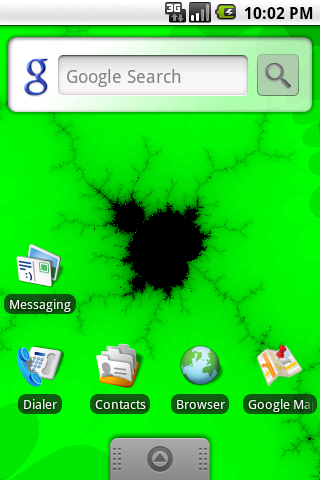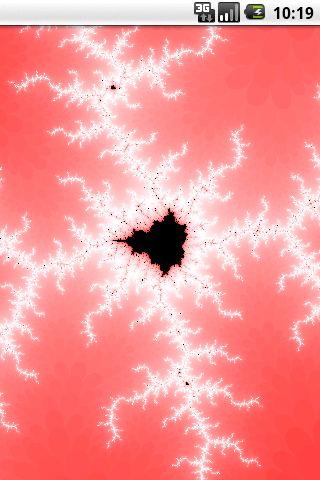# Mandelbrot Set for Android

 Using saved image as wallpaper Changing colors[How to control]
• By pintching the screen, the set is expanded.

• By pressing the menu button, you can perform the following controls.
• Resize : The default state is restored.
• Change color : You can select the color from four color modes.
• Magnify rate: You can change the expansion rate.
• Save image : You can save image.
[Description]

Let us consider a map in the complex plane written as
z n+1 = zn2 + c.

Depending on the value of complex parameter c, the trajectory zn from the initial condition z0=0 converges to fixed points, periodic orbits, or chaotic orbits, but with some values of parameter c, zn diverges to the infinity.

The set of parameter c for which the trajectory does not diverge is called as Mandelbrot set.
This simulator shows the Mandelbrot set in the complex plane.
The set is colored with black, and the points which do not belong to it are colored depending on the time to diverge.

When the simulator is expanded with your mouse, Mandelbrot set shows infinitely fine structures.
Dr. Mandelbrot named such a structure with self-similarity as fractal.

In the later researches, Dr. M. Shishikura mathematically proved that the dimension of the boundary of the Mandelbrot set is 2, and it is connective.
In many cases, the fractal figures have non-integer dimensions, thus the above proof means that the Mandelbrot set is not an typical example of fractal.
However, the impact which Mandelbrot set gives to the researches on fractal and chaos is large,
thus it is often introduced as a monumental diagram even now.